Ex 11.1

Chapter 11 Class 10 Constructions [Deleted]
Serial order wise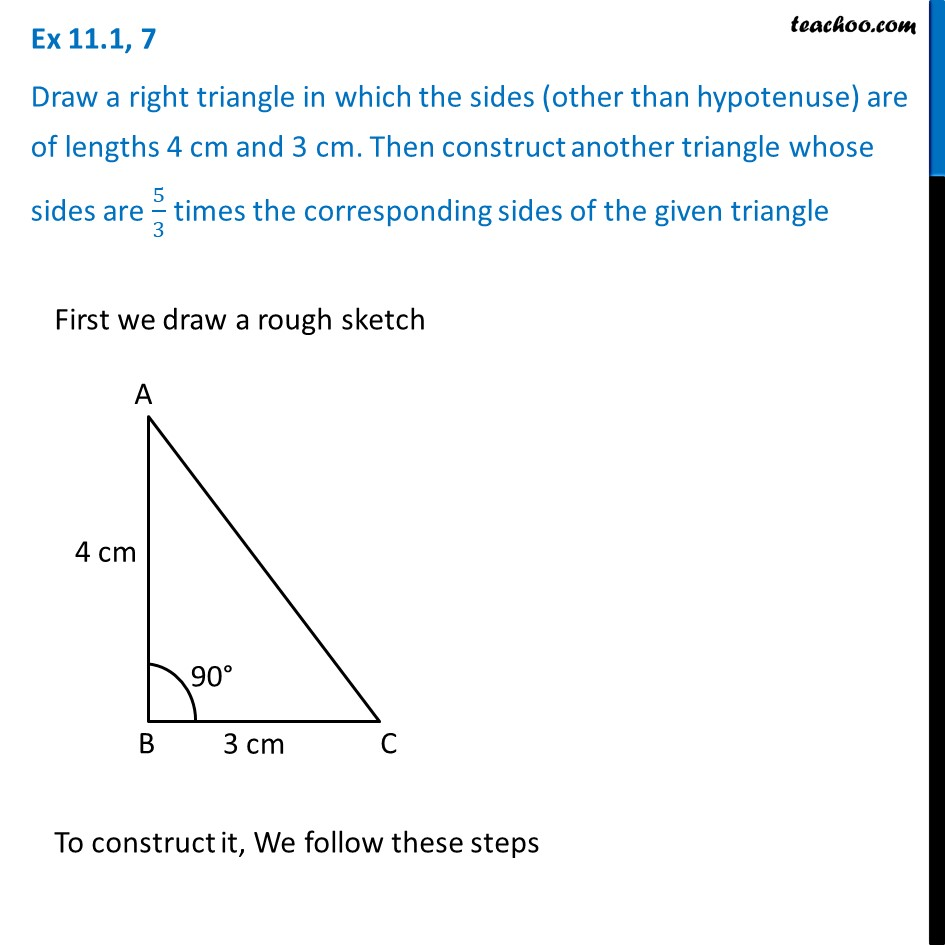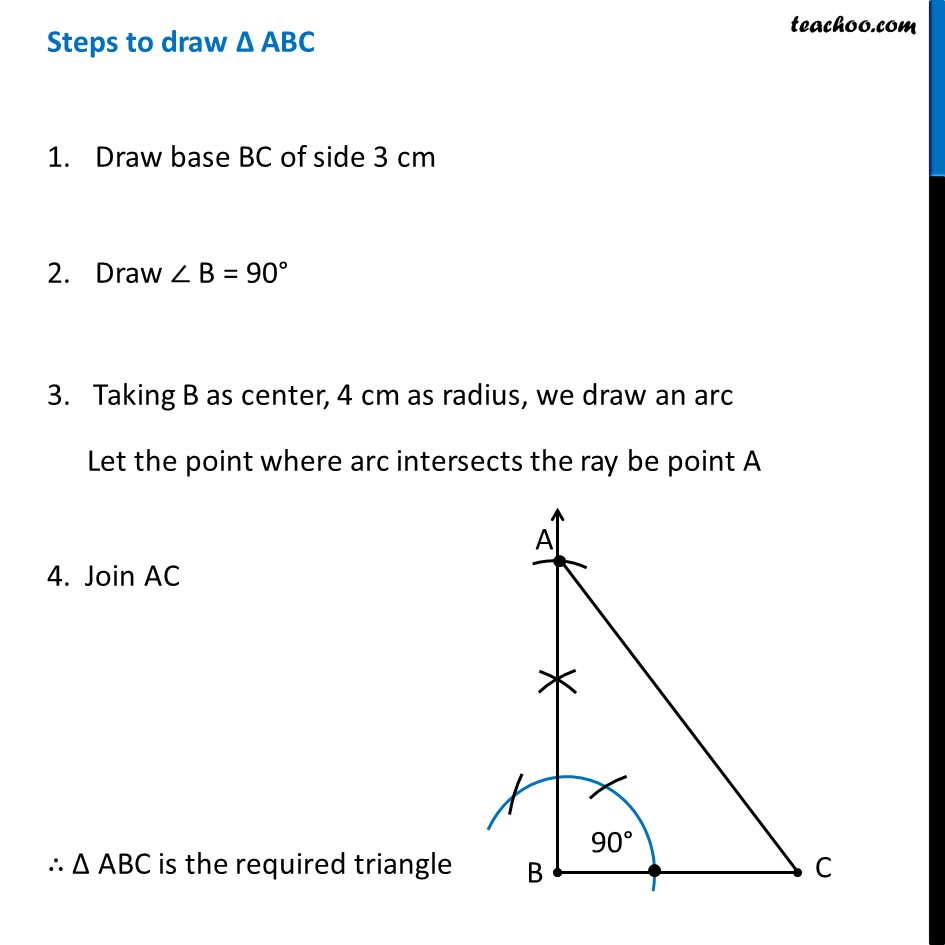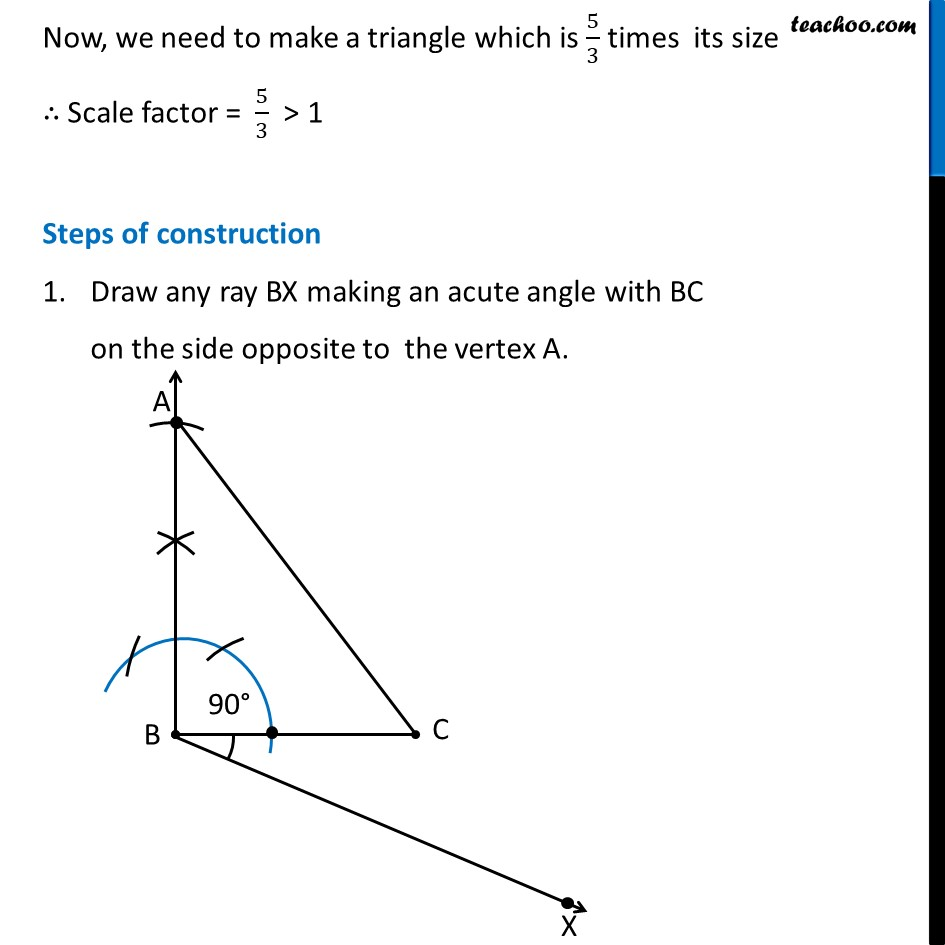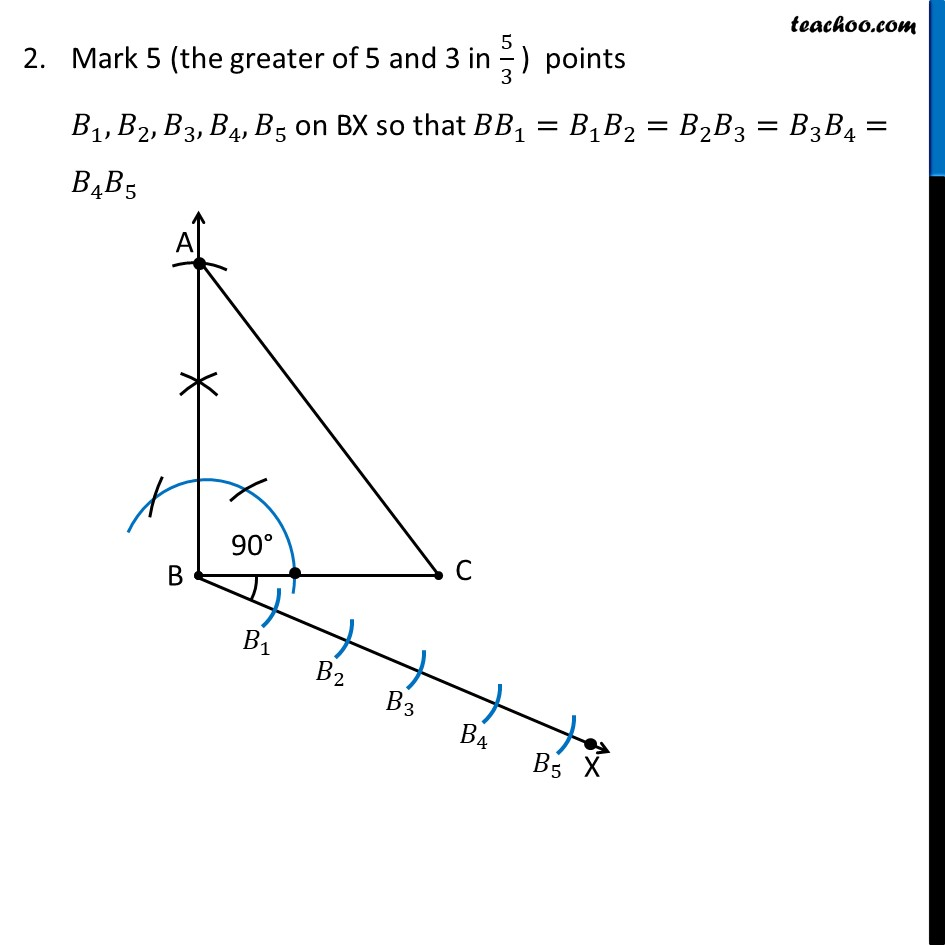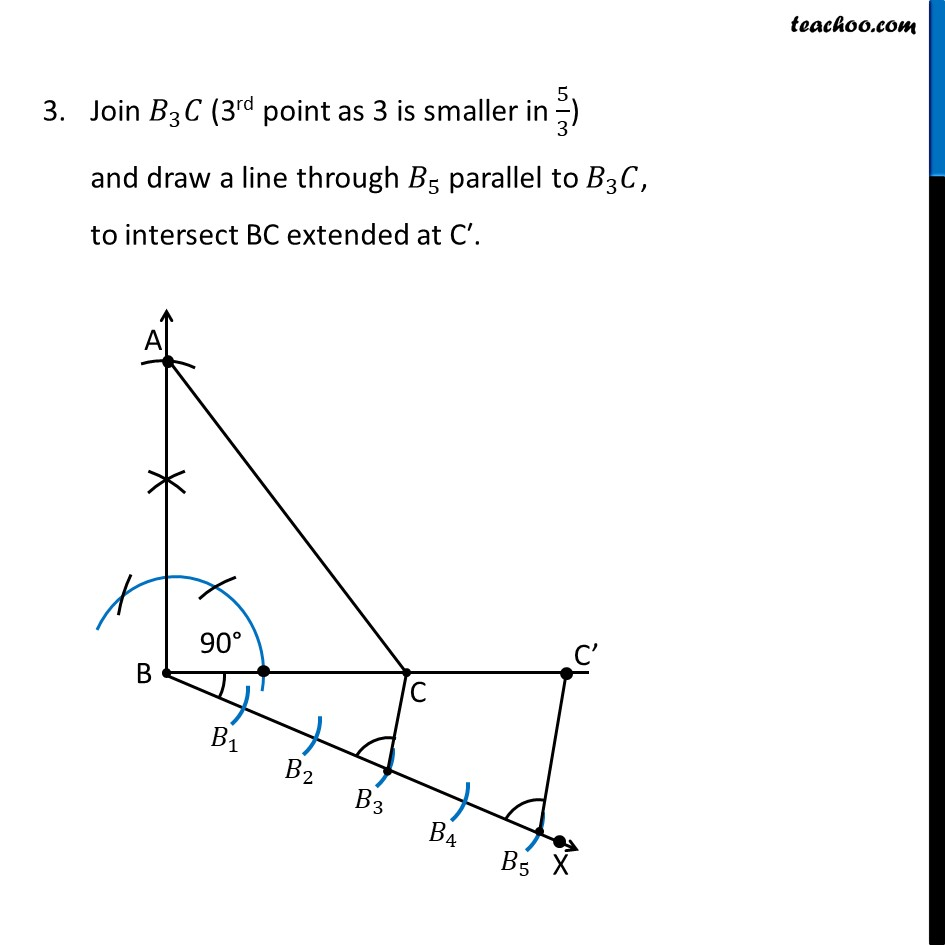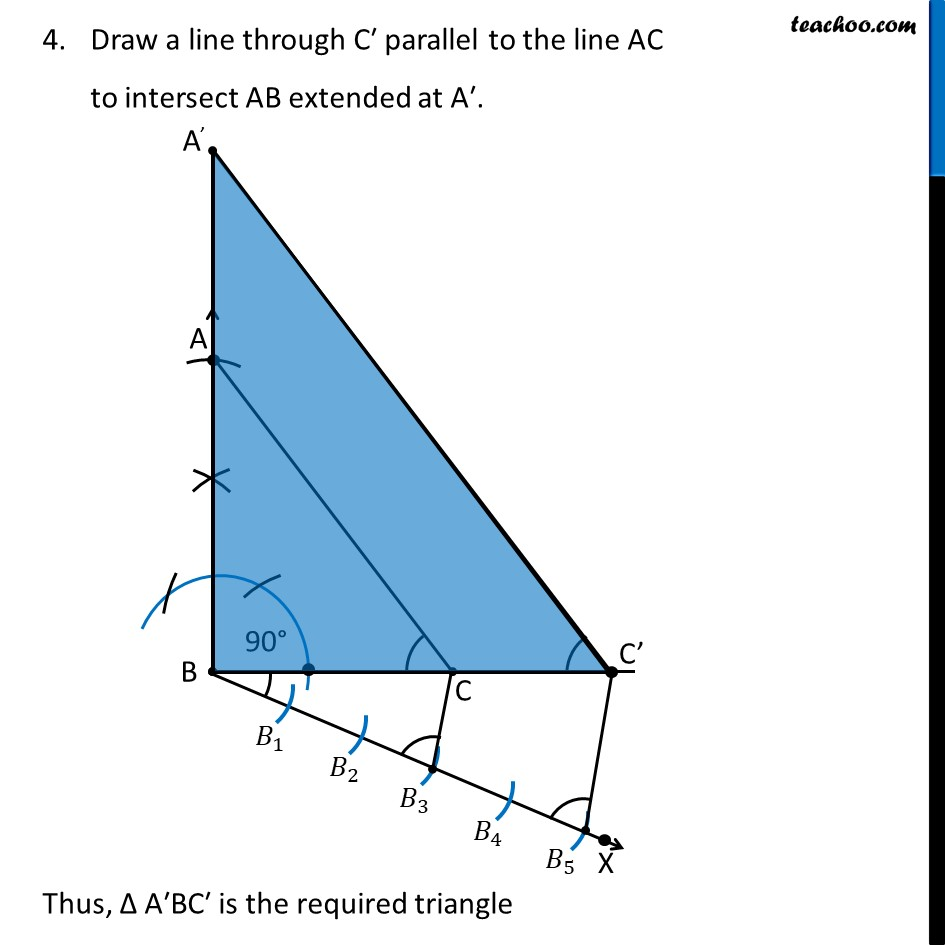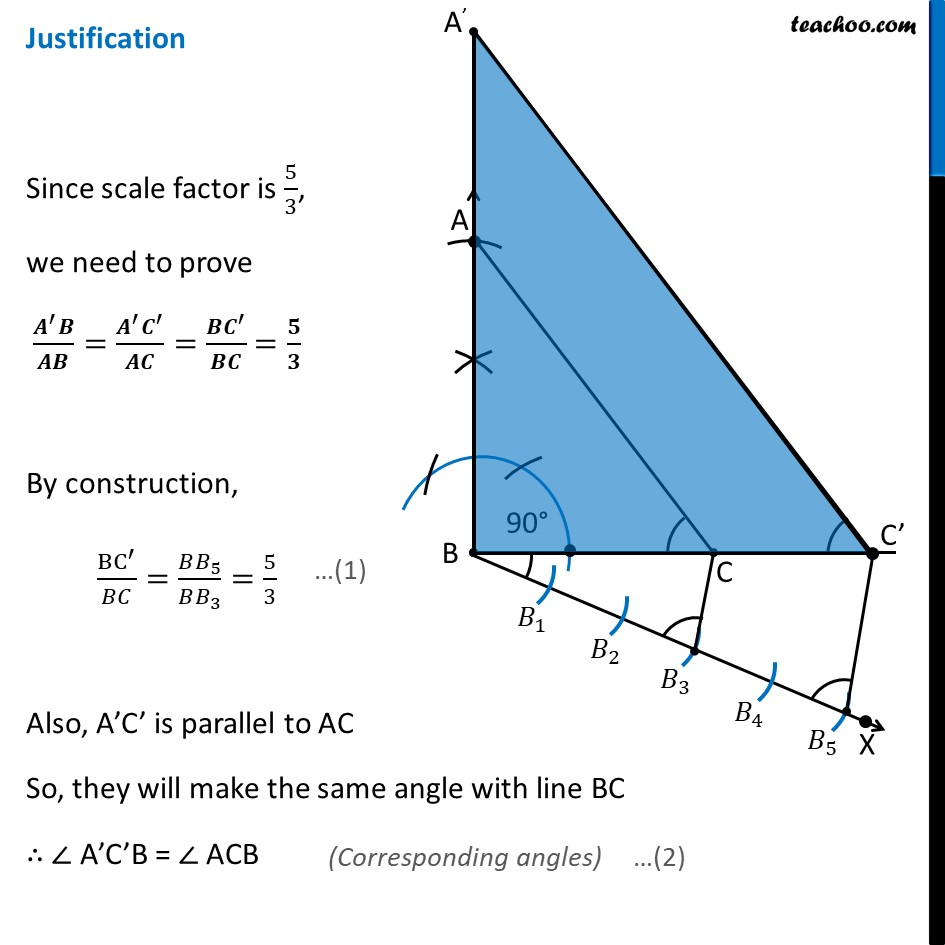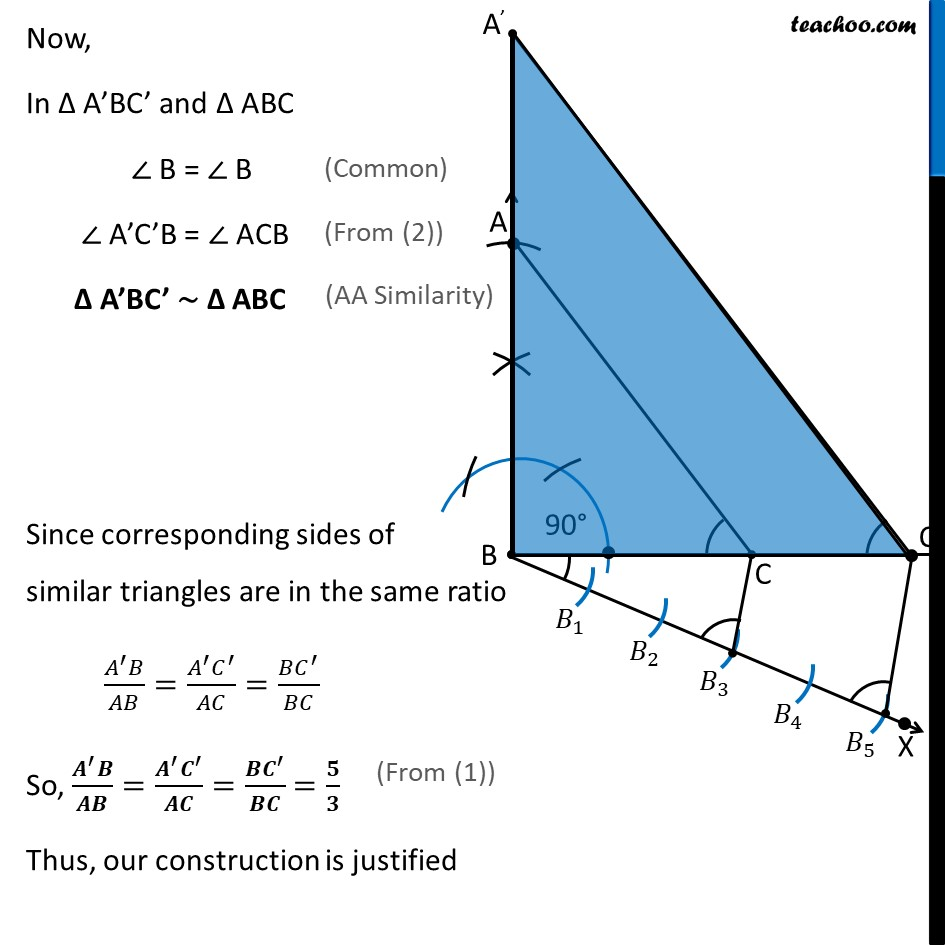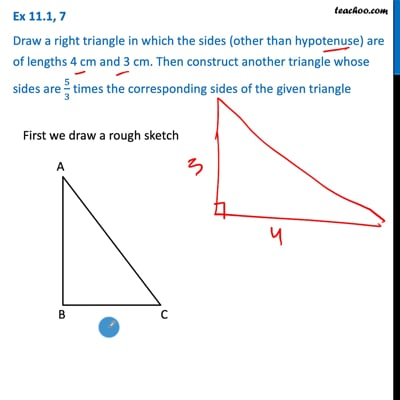This video is only available for Teachoo black users

Maths Crash Course - Live lectures + all videos + Real time Doubt solving!

### Transcript

Ex 11.1, 7 Draw a right triangle in which the sides (other than hypotenuse) are of lengths 4 cm and 3 cm. Then construct another triangle whose sides are 5/3 times the corresponding sides of the given triangle First we draw a rough sketch To construct it, We follow these steps Steps to draw Δ ABC Draw base BC of side 3 cm Draw ∠ B = 90° 3. Taking B as center, 4 cm as radius, we draw an arc Let the point where arc intersects the ray be point A 4. Join AC ∴ Δ ABC is the required triangle Now, we need to make a triangle which is 5/3 times its size ∴ Scale factor = 5/3 > 1 Steps of construction Draw any ray BX making an acute angle with BC on the side opposite to the vertex A. Mark 5 (the greater of 5 and 3 in 5/3 ) points 𝐵_1, 𝐵_2, 𝐵_3,𝐵_4,𝐵_5 on BX so that 〖𝐵𝐵〗_1=𝐵_1 𝐵_2=𝐵_2 𝐵_3=𝐵_3 𝐵_4=𝐵_4 𝐵_5 Join 𝐵_3 𝐶 (3rd point as 3 is smaller in 5/3) and draw a line through 𝐵_5 parallel to 𝐵_3 𝐶, to intersect BC extended at C′. Draw a line through C′ parallel to the line AC to intersect AB extended at A′. Thus, Δ A′BC′ is the required triangle Justification Since scale factor is 5/3, we need to prove (𝑨^′ 𝑩)/𝑨𝑩=(𝑨^′ 𝑪^′)/𝑨𝑪=(𝑩𝑪^′)/𝑩𝑪 =𝟓/𝟑 By construction, BC^′/𝐵𝐶=(𝐵𝐵_5)/(𝐵𝐵_3 )=5/3 Also, A’C’ is parallel to AC So, they will make the same angle with line BC ∴ ∠ A’C’B = ∠ ACB Now, In Δ A’BC’ and Δ ABC ∠ B = ∠ B ∠ A’C’B = ∠ ACB Δ A’BC’ ∼ Δ ABC Since corresponding sides of similar triangles are in the same ratio (𝐴^′ 𝐵)/𝐴𝐵=(𝐴^′ 𝐶^′)/𝐴𝐶=(𝐵𝐶^′)/𝐵𝐶 So, (𝑨^′ 𝑩)/𝑨𝑩=(𝑨^′ 𝑪^′)/𝑨𝑪=(𝑩𝑪^′)/𝑩𝑪 =𝟓/𝟑 Thus, our construction is justified